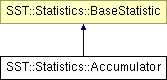# SST::Statistics::Accumulator Class Reference

Allows the online gathering of statistical information about a single quantity. More...

`#include <accumulator.h>`

Inheritance diagram for SST::Statistics::Accumulator:## Public Member Functions

Accumulator (char *name)
Create a new Accumulator class with initial values set to a zero count, zero sum statistic of interest.
NumberBase getSum ()
Provides the sum of the values presented so far.
NumberBase getSumSquared ()
Provides the sum of each value squared presented to the class so far.
void add (NumberBase value)
Present a new value to the class to be included in the statistics.
void add (NumberBase *values, uint32_t length)
Present an array of values to the class to be included in the statistics.
NumberBase getArithmeticMean ()
Get the arithmetic mean of the values presented so far.
NumberBase getVariance ()
Get the variance of the values presented so far.
NumberBase getStandardDeviation ()
Get the standard deviation of the values presented so far.
uint64_t getCount ()
Get a count of the number of elements presented to the statistics collection so far.

## Detailed Description

Allows the online gathering of statistical information about a single quantity.

The basic statistics are captured online removing the need to keep a copy of the values of interest.

Template Parameters:
 NumberBase A template for the basic numerical type of values

## Member Function Documentation

 void SST::Statistics::Accumulator::add ( NumberBase * values, uint32_t length ) ` [inline]`

Present an array of values to the class to be included in the statistics.

Parameters:
 values The array of values to be added to the statistics collection length The length of the array being presented
 void SST::Statistics::Accumulator::add ( NumberBase value ) ` [inline]`

Present a new value to the class to be included in the statistics.

Parameters:
 value New value to be presented
 NumberBase SST::Statistics::Accumulator::getArithmeticMean ( ) ` [inline]`

Get the arithmetic mean of the values presented so far.

Returns:
The arithmetic mean of the values presented so far.
 uint64_t SST::Statistics::Accumulator::getCount ( ) ` [inline]`

Get a count of the number of elements presented to the statistics collection so far.

Returns:
Count the number of values presented to the class.
 NumberBase SST::Statistics::Accumulator::getStandardDeviation ( ) ` [inline]`

Get the standard deviation of the values presented so far.

Returns:
The standard deviation of the values presented so far

References getVariance().

 NumberBase SST::Statistics::Accumulator::getSum ( ) ` [inline]`

Provides the sum of the values presented so far.

Returns:
The sum of values presented to the class so far.
 NumberBase SST::Statistics::Accumulator::getSumSquared ( ) ` [inline]`

Provides the sum of each value squared presented to the class so far.

Returns:
The sum of squared values presented to the class so far.
 NumberBase SST::Statistics::Accumulator::getVariance ( ) ` [inline]`

Get the variance of the values presented so far.

Returns:
The variance of the values presented so far

Referenced by getStandardDeviation().

The documentation for this class was generated from the following file:

Generated on 14 Sep 2015 for SST by1.6.1